This is the first in a series of blog posts I'm going to write around understanding DAX and Power BI!

There is a central idea in DAX – an evaluation context. This underpins the way in which the entire language works, so I think it's important to get your head around first. Any formula you input will define computational logic that will be run in an evaluation context. The output of that formula will depend on the data set that the formula is run over.

The rows which are present in that dataset is called the filter context.

The filter context is made up of a combination of factors. The most obvious one in Power BI is any filter which has been placed on the report as a whole (usually via the visual tools). Any expressions which are evaluated on the pages of the report will be executed over a dataset which has been reduced using those filters (if anyone is wanting to shout at me about `ALL` or `CALCULATE` right now, bear with me).

So, if we had the following dataset: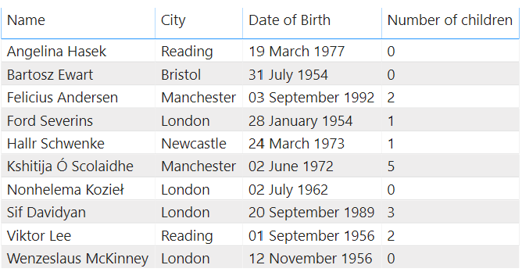We can calculate the total number of children using `SUM(People[Number of children])`: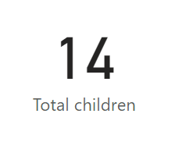If we then apply a filter to the report, so that we are only looking at the people who live in London: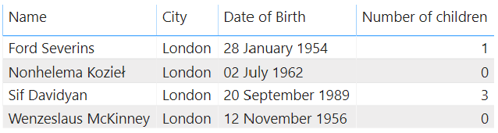This is then a new filter context: `People[City] = "London"`. This context will be used to evaluate any formulae we have entered, and therefore the `SUM` of children will only operate over the reduced dataset, and the output of the total children formula will change: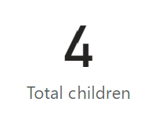This seems fairly simple, but the idea that there is a filter context present whenever any expression is evaluated is crucial to understanding how DAX works.

There are other factors which can contribute to a filter context. If we remove our report filters and build a table view of the data where we can see the number of children by city: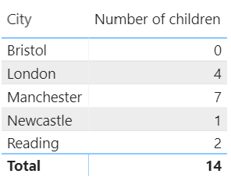This is a table which has aggregated the total number of children for each city.

Each row of the table is calculated using a different filter context. The first row us calculated using a `SUM` where the applied filter context is `People[City] = "Bristol"`, this means that the raw dataset has been reduced to the rows for which the city is Bristol, and the SUM has then been run over these rows. The second row of this visual is done by scanning the dataset produced by `People[City] = "London"`, etc. The total is then calculated not by summing the individual rows but by doing another scan of the entire table but with an empty filter context.

In this way we can see that each row of the aggregated table is applying a filter context. If we had separate columns for male and female children, then we could have additional columns in this table which again would each have their own filter context under which to do the aggregation.

So we have four ways in Power BI in which to define a filter context:

• Row selection
• Column selection
• Slicer selection
• Report/visual filters

There is another type of evaluation context – a row context but that is a subject for next time!

### Carmel Eve

#### Software Engineer I